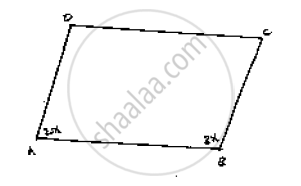# Two Unequal Angles of a Parallelogram Are in the Ratio 2 : 3. Find All Its Angles in Degrees . - Mathematics

Two unequal angles of a parallelogram are in the ratio 2 : 3. Find all its angles in degrees .

#### SolutionLet ∠A = 2x and ∠B = 3x

Now,

∠A + ∠B = 180°                           [Co-interior angles are supplementary]

2x + 3x -180°                              [ AD || BC and AB is the transversal]

⇒ 5x =180°

⇒ x = (180°)/ 5 = 36°

∴∠A = 2 ´ 36° = 72°

∠ B = 3´ 36° = 108°

Now,

∠A = ∠C = 72°                           [Opposite side angles of a parallelogram are equal]

∠B = ∠D =108°

Concept: Pairs of Angles
Is there an error in this question or solution?

#### APPEARS IN

RD Sharma Mathematics for Class 9
Chapter 10 Lines and Angles
Exercise 10.4 | Q 7 | Page 47